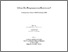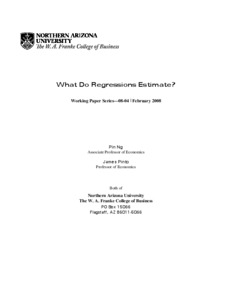# What do regressions estimate? Working paper series--08-04

Ng, Pin and Pinto, James (2008) What do regressions estimate? Working paper series--08-04. Working Paper. NAU W.A. Franke College of Business.Preview
Text
Ng_P_Etal_2008_FrankeWPS_08-04(1).pdf

## Abstract

Along with the readily available computer software that performs least squares regressions, comes the proliferation of misuse and misinterpretation of the regression results. We illustrate in the paper that the solutions to the intercept and slope coefficients in a simple linear regression model are dependent on the definition of the loss function used in the optimization problem. Likewise, there is not a universal population linear regression line. If a squared error loss function is used, the population regression line turns out to be the conditional mean function while an absolute loss function yields the conditional median. When the asymmetric loss function defined in Koenker and Bassett (1978) is used, the population regression line becomes the conditional quantile function. Therefore, what the sample regression is estimating and how the estimated intercept and slope coefficients should be interpreted are dependent upon what loss function is being used in the optimization problem. We illustrated a few mistakes commonly made in interpreting the least squares regression results and discuss some potential misuses of the least square regressions. By pointing out these specific mistakes, we hope that practitioners and educators will have a better understanding of what exactly we are estimating when we perform a least squares regression or any regression for that matter.

Item Type: Monograph (Working Paper) Copyright, where appropriate, is held by the author. 08-04 Working paper, linear regression, least squares, population, interpretation of statistical results H Social Sciences > HA StatisticsH Social Sciences > HF Commerce Faculty/Staff The W.A. Franke College of Business 18 Oct 2015 00:47 http://openknowledge.nau.edu/id/eprint/1531IR Staff Record View